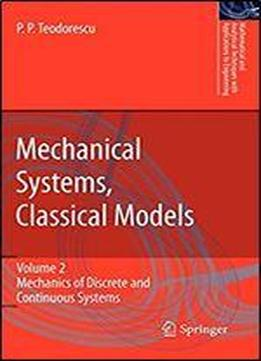# Mechanical Systems, Classical Models: Volume Ii: Mechanics Of Discrete And Continuous Systems (mathematical And Analytical Techniques With Applications To Engineering) by Petre P. Teodorescu / 2008 / English / PDF

As it was already seen in the first volume of the present book, its guideline is precisely the mathematical model of mechanics. The classical models which we refer to are in fact models based on the Newtonian model of mechanics, on its five principles, i. e. : the inertia, the forces action, the action and reaction, the parallelogram and the initial conditions principle, respectively. Other models, e. g. , the model of attraction forces between the particles of a discrete mechanical system, are part of the considered Newtonian model. Kepler’s laws brilliantly verify this model in case of velocities much smaller than the light velocity in vacuum. The non-classical models are relativistic and quantic. Mechanics has as object of study mechanical systems. The first volume of this book dealt with particle dynamics. The present one deals with discrete mechanical systems for particles in a number greater than the unity, as well as with continuous mechanical systems. We put in evidence the difference between these models, as well as the specificity of the corresponding studies the generality of the proofs and of the corresponding computations yields a common form of the obtained mechanical results for both discrete and continuous systems. We mention the thoroughness by which the dynamics of the rigid solid with a fixed point has been presented. The discrete or continuous mechanical systems can be non-deformable (e. g.

views: 226